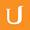## Bunnies Solution - Intro to Computer Science

• 0:01 - 0:02
So here's one way to solve this, we're
• 0:02 - 0:05
going to define a procedure Fibonacci and we'll call the
• 0:05 - 0:07
input n. And now we need to write
• 0:07 - 0:10
the code, and if we remember our definitions; so
• 0:10 - 0:15
we said Fibonacci of 0 is defined as 0,
• 0:15 - 0:21
Fibonacci of 1 is defined as 1. And Fibonacci of any higher number
• 0:21 - 0:26
is defined as Fibonacci of n minus 1 plus Fibonacci of n
• 0:26 - 0:29
minus 2. So if you remember the definition, we
• 0:29 - 0:32
have two base cases we need to consider. If the
• 0:32 - 0:34
input value is 0, or the input value is 1,
• 0:34 - 0:37
we need to do something special. So we could write
• 0:37 - 0:41
those as separate if statements. So if n is
• 0:41 - 0:44
equal to 0, what we want to do is return
• 0:44 - 0:51
0. If n is equal to 1, what we want to do is return 1. Otherwise what we want to
• 0:51 - 0:56
do is the recursive part of the definition. So we want
• 0:56 - 1:01
to return the result of Fibonacci n minus 1, and we want
• 1:01 - 1:05
to add that to Fibonacci n minus 2. So we could
• 1:05 - 1:08
simplify this a little bit. Let's try this in the Python interpreter.
タイトル：
Bunnies Solution - Intro to Computer Science

more » « less
Video Language:
English
Team:Udacity
プロジェクト：
CS101 - Intro to Computer Science
Duration:
01:10Udacity Robot edited 英語(米国) subtitles for 22-19 Bunnies SolutionUdacity Robot edited 英語(米国) subtitles for 22-19 Bunnies SolutionUdacity Robot edited 英語(米国) subtitles for 22-19 Bunnies SolutionCogi-Admin edited 英語(米国) subtitles for 22-19 Bunnies Solution

# English subtitles

## 改訂 Compare revisions

• API
Udacity Robot
• API
Udacity Robot
• API
Udacity Robot
• API